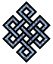#fail2ban bad ip database: ip 80.21.147.85

### | ip database | live view | stats | report | help | api key:

 ip: 80.21.147.85 hostname: host85-147-static.21-80-b.business.telecomitalia.it country:[IT] Italy first reported: 20.10.2018 02:43.09 GMT+0200 last reported: 22.04.2019 03:12.36 GMT+0200 time period: 184d 29m 27s total reports: 6 reported by: 3 host(s) filter(s): ssh (6) tor exit node no badips.com db Lookup## port scan of '80.21.147.85':

[-hide]
```# Nmap 6.40 scan initiated Sat Oct 20 02:44:01 2018 as: /usr/bin/nmap -sU -sS -O 80.21.147.85
Nmap scan report for host85-147-static.21-80-b.business.telecomitalia.it (80.21.147.85)
Host is up (0.053s latency).
Not shown: 994 filtered ports, 993 closed ports
PORT      STATE         SERVICE
22/tcp    open          ssh
23/tcp    open          telnet
1723/tcp  open          pptp
7/udp     open          echo
9/udp     open|filtered discard
19/udp    open          chargen
49/udp    open|filtered tacacs
67/udp    open|filtered dhcps
68/udp    open|filtered dhcpc
161/udp   open          snmp
162/udp   open|filtered snmptrap
1701/udp  open|filtered L2TP
21476/udp open|filtered unknown
No exact OS matches for host (If you know what OS is running on it, see http://nmap.org/submit/ ).
TCP/IP fingerprint:
OS:SCAN(V=6.40%E=4%D=10/20%OT=22%CT=1%CU=%PV=N%G=Y%TM=5BCA85A5%P=x86_64-pc-
OS:linux-gnu)SEQ(SP=107%GCD=1%ISR=10E%TI=Z%CI=RD%TS=U)OPS(O1=M5B4%O2=M5B4%O
OS:3=M5B4%O4=M5B4%O5=M5B4%O6=M5B4)WIN(W1=3908%W2=3908%W3=3908%W4=3908%W5=39
OS:08%W6=3908)ECN(R=Y%DF=Y%TG=40%W=3908%O=M5B4%CC=Y%Q=)T1(R=Y%DF=Y%TG=40%S=
OS:O%A=S+%F=AS%RD=0%Q=)T2(R=N)T3(R=N)T4(R=Y%DF=Y%TG=40%W=0%S=A%A=Z%F=R%O=%R
OS:D=0%Q=)T5(R=Y%DF=N%TG=FF%W=0%S=A%A=S+%F=AR%O=%RD=0%Q=)T6(R=Y%DF=N%TG=FF%
OS:W=0%S=A%A=Z%F=R%O=%RD=0%Q=)T7(R=Y%DF=N%TG=FF%W=0%S=A%A=S%F=AR%O=%RD=0%Q=
OS:)U1(R=N)IE(R=Y%DFI=S%TG=FF%CD=S)

OS detection performed. Please report any incorrect results at http://nmap.org/submit/ .
# Nmap done at Sat Oct 20 03:32:21 2018 -- 1 IP address (1 host up) scanned in 2899.96 seconds
```
```Σ = 114 | Δt = 0.0053210258483887s
```